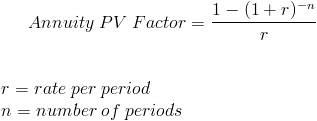## Present Value of Annuity Due:

Present value of annuity due is given as the sum of the present values of the individual cash flows in an equal periodic series of cash flows from the beginning of a period.

Jack should choose the option with the higher present value.

For Alternative 1

Present value = $44,000,000.00 For Alternative 2 Present value = Annuity + Annuity * PVAF (r, (n - 1)) PVAF or present value annuity factor is the sum of discounting factors at a given rate r for n number of years. It can be calculated using the following formula:Here, • Annuity =$2,900,000.00
• r = 5% = 0.05
• n = 30

So, Present value
= 2,900,000 + 2,900,000 x PVAF (5%,30 years)
= 2,900,000 + 2,900,000 x 15.3725
= \$47,480,250.00

Jack should choose the second alternative as it gives the higher present value.